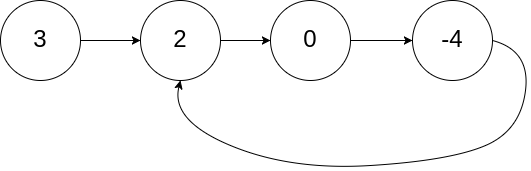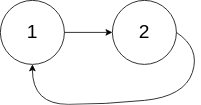# 面试题 02.08 环路检测### 面试题 02.08. 环路检测

Difficulty: 中等``````输入：head = [3,2,0,-4], pos = 1

````````````输入：head = [1,2], pos = 0

````````````输入：head = , pos = -1

``````

• 你是否可以不用额外空间解决此题？

#### Solution

Language: c++

• 关系 1: `f = 2s;` 注: f 为 快指针的路程, s 为满指针的路程
• 关系 2: `任何指针如果要走到 环的起点, 那么肯定需要走的距离 = a + nb; 其中 a 为环外的节点数; b 为环的节点数, n 为自然数`
• 关系 3: 第一次相遇的时候, s 从环的起点走过的结束数 为 k
• 此时 `s = a + k;` `f = a + k + nb;` => 推导出关键点: `s = nb;`
• 根据关系 2可以推导出 此时从 相遇点, 再走 a 步, 肯定就走到了 环的起点了;
• 那么 f 走 a 步 (相遇点),同时 s 从链表的起点再走 a 步, 显然就相遇了. 环的起点就知道了.
``````/**
* struct ListNode {
*     int val;
*     ListNode *next;
*     ListNode(int x) : val(x), next(NULL) {}
* };
*/

class Solution {
public:
while (fast != NULL && fast->next != NULL){
fast = fast->next->next;
slow = slow->next;
if (slow == fast) {